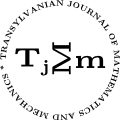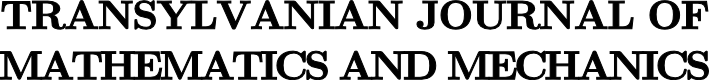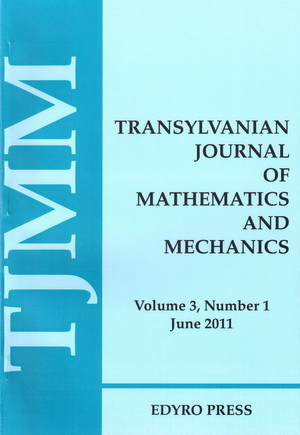ISSN: 2067-239X
ISSN(on-line): 2067-239X

Indexed in:
Mathematical Reviews
Zentralblatt MATH
EBSCO

Front cover# Volume 4 (2012), Number 2

## A Generalization of Companion Inequality of Ostrowski's Type for Mappings Whose First Derivatives Are Bounded and Applications in Numerical Integration

Abstract: An inequality for a companion of Ostrowski's integral inequality is proved. Application to a composite quadrature rule is considered.

## Growth of Universal Entire Harmonic Functions

### Author(s): DEVENDRA KUMAR AND SACHIN KUMAR GUPTA

Abstract: In this paper we obtained some bounds on growth parameters order and type of $J$ -universal entire harmonic functions on ${ℝ}^{N}$. The results are expressed in terms of the derivatives of function at origin on ${ℝ}^{N}$.

## A Class of Nonlinear Integral Equations

### Author(s): MARIA DOBRIȚOIU

Abstract: Using the Contraction Principle and the General Data Dependence Theorem, several results of existence and uniqueness and of continuous dependence of data of the solution of a class of integral equations with modified argument from physics, that is a mathematical model reference with to the turbo-reactors working: $x\left(t\right)={\int }_{a}^{b}K\left(t,s\right)·h\left(s,x\left(s\right),x\left(a\right),x\left(b\right)\right)ds+f\left(t\right),t\in \left[a,b\right],$ where $a,b\in ℝ$, $a, $K:\left[a,b\right]×\left[a,b\right]\to ℝ$, $h:\left[a,b\right]×{ℝ}^{3}\to ℝ$, $f:\left[a,b\right]\to ℝ$ and $x:\left[a,b\right]\to ℝ$ are given. Also, an example is given.

## New Ostrowski Type Inequalities for Co-ordinated Convex Functions

### Author(s): MUHAMMAD AMER LATIF, SABIR HUSSAIN AND SEVER S. DRAGOMIR

Abstract: In this paper some new Owstroski type inequalites for co-ordinated convex functions are obtained.